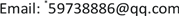1. 前言

2. PEMFC系统2.1. 系统模型的设计

PEMFC系统主要包括供气系统、电池系统、冷却系统、尾气回收系统。本文设计的PEMFC系统模型可见图1。

2.2.1. PEMFC模块

V c e l l = E N e r s t − V a c t − V o h m − V c o n (2-1)

1) 热力学电动势

(2-2)

E N e r s t = 1.229 − 8.5 × 10 − 4 × ( T − 298.15 ) + 4.308 × 10 − 5 T × ( ln P H 2 + 0.5 ln P O 2 ) (2-3)

2) 活化过电势

{ V a c t = δ 1 + σ T + δ 3 T ln C O 2 + δ 4 T ln I σ = δ 2 + 2 × 10 − 4 ln A + 4.3 × 10 − 5 ln C H 2 C O 2 = 1.97 × 10 − 7 × P O 2 × exp ( 498 / T ) C H 2 = 9.17 × 10 − 7 × P H 2 × exp ( − 77 / T ) (2-5)

3) 欧姆过电势

(2-6)

4) 浓差过电势

PEMFC燃料电池在反应过程中需要燃料与氧化剂，并且在会生成一定量的水。这就会导致催化层内的生成物与反应物的浓度会发生变化，这就会产生浓差损失影响电池的输出性能。

V c o n = − m exp ( n i ) (2-7)

2.2.2. 其余子模块

1) 高压压气机

π = f 1 ( G i n P 0 T i n P i n T 0 , n c T 0 T i n ) (2-8)

(2-9)

N e C = c p a T 1 ( π m a − 1 ) / η (2-10)

m a = ( λ a − 1 ) / λ a (2-11)

T 2 = T 1 [ 1 + ( π m a − 1 ) / η ] (2-12)

2) 管道模型

(2-13)

d P s m d t = γ R a V s m ( F c p T c p , out − F s m , out T s m ) (2-14)

F s m , out = k s m , out ( P s m − P c a ) (2-15)

d p r m d t = R a T r m V r m ( F c a , out − F r m ) (2-16)

F r m = C D , r m A T , r m p r m R T r m γ 1 2 ( 2 γ + 1 ) γ + 1 2 ( γ − 1 ) ， p a t m p r m ≤ ( 2 γ + 1 ) γ γ − 1 (2-17)

3) 涡轮模型

(2-18)

4) 阴极空气动态压力模型

d m O 2 d t = F O 2 , in − F O 2 , out − F O 2 , r e c (2-19)

d m N 2 d t = F N 2 , in − F N 2 , out (2-20)

F O 2 , in = 0.21 M O 2 M a i r ⋅ F s m , out 1 + Ω atm (2-21)

F O 2 , in = 0.79 M N 2 M a i r ⋅ F s m , out 1 + Ω atm (2-22)

P N 2 = n N 2 R N 2 T V c a (2-24)

P c a = P O 2 + P N 2 (2-25)

(2-26)

F N 2 , out = m N 2 m c a F c a , out (2-27)

m c a = m O 2 + m N 2 (2-28)

F O 2 , r e c = M O 2 n I 4 F (2-29)

5) 废气利用率

3. 仿真结果分析3.1. 仿真分析

3.2. 探究影响PEMFC输出性能的因素

1) 温度对PEMFC输出性能的影响

2) 氧气过量系数对PEMFC输出性能的影响

4. 结论